# We Helped With This MATLAB Programming Homework: Have A Similar One?

SOLVEDCategory Programming MATLAB College Solved Best Matlab Assignment Help

## Assignment DescriptionMECH 529 – Laboratory 11

“Optimization of Dynamic Systems”

(970) 491-3539, ...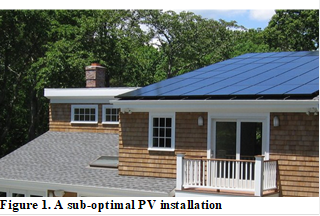Introduction:

All last week we have been talking about methods of optimization of dynamic systems.  We now get the opportunity to apply that knowledge to our models.  Our application will be the power production of photovoltaic panels.

Learning Objectives:

1)     Test a number of the computer modeling techniques that we have learned in the ME529 laboratory with the construction of an model of a nonlinear dynamic (thermal) system

2)     Introduce penalty functions as a means for imposing constraint on system optimization algorithms

3)     Use optimization to define ideal conditions of operation for a dynamic system

Many optimization routines (including Nelder Mead simplex) that are used for system design are unconstrained optimization routines.  To add constraints on the variables (that are changing during the optimization) requires an augmentation of the cost function.  One way to accomplish that is penalty functions.

As an example, let us assume that you would like to conduct an optimization for the problem:

Minimize f(x)=-2x, subject to x<5

The problem is that Nelder-Mead has no way to incorporate your constraint on the admissible values of x.  You must augment the cost function f(x) with a penalty function that communicates violation of the constraint to the solver by increasing the cost function when the constraint is violated.  In this case, you can use a penalty function of the form:

p(x) = rp*(max(0,x-5))2

The variable rp r is a variable that determines the size of the penalty that is applied when the constraint is violated.  As rp increases, violation of the constraints is more and more penalized.  Often you will change rp in subsequent optimization operations to assure that the constraints are not violated.  Addition of this penalty function to the cost function results in an augmented cost function of:

f(x)=2x + rp*(max(0,x-5))2

To construct a penalty function is easy for inequality constraints: put the constraint equation into the form of an equation that is less than zero (g(x)<0) and use rp*max(0,g(x))2 as the penalty function.  To construct a penalty function for equality constraints, put the constraint in the form of g(x)=0, and use the penalty function rp*g(x)2.  Try to construct a penalty function with the following constraints:

x=4

-x > 3

x1 <x2+5

One thing to note is that in order for the penalty functions to be effective, they must be of the same order of magnitude as each other and of the same order of magnitude (or greater) as the cost function.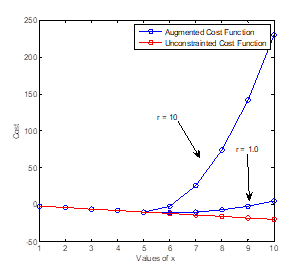Figure 2. Comparison of augmented and conventional cost function for the
external penalty method example problem, r=10

MATLAB™’s Nelder Mead n-dimensional simplex method is called fminsearch.  To do an example problem with fminsearch,

Minimize         f = -x1x2

Subject to       g1 = x1+x22-1 < 0

g2 = -x1-x2 <0

To solve this problem you will have to make two m-files.  One will be a script file that you use to call fminsearch.  The other will be a function that evaluates f as a function of x1 and x2.  I pass the vector of x1 and x2 to the evaluation function along with the value of the penalty, r.  Here is the code for the script file.  I have varied r so that the constraints are:

clear

close all

optim_options = optimset('Display','iter');

[x1,x2] = meshgrid(-1:.1:1, -1:.1:2);

index = 0;

for rp = [1:10:31]

close all

index=index+1;

f_const = -x1.*x2 + rp*max(0,x1+x2.^2-1).^2 + rp*max(0,-x1-x2).^2;

f_unconst = -x1.*x2 ;

subplot(1,2,1);

contourf(x1,x2, f_unconst,[-.5 -.4 [0:.5:5]]); title(' Unconstrained Problem'); colorbar

subplot(1,2,2); contourf(x1,x2, f_const,[-.5 -.4 [0:.5:5]]); hold on ; colorbar

plot([-1:.01:1],sqrt(-[-1:.01:1]+1),'k'); plot([-1 1], [1 -1],'k')

title(['Find Optimum Between the 2 Black Lines, Rp = ' num2str(rp)])

[x] = fminsearch(@(x) example_fxn(x,rp),[-.5 -.5],optim_options)

Solution(index,:)=x;

end

Here is the code for the function.  You can see that I have put the penalty functions in the evaluation of the cost function f:

function f = example_fxn(x,rp)

pause(.5)

f = -x(1)*x(2) + rp*max(0,x(1)+x(2)^2-1)^2 + rp*max(0,-x(1)-x(2))^2;

plot(x(1), x(2),'ro-');

Run the example and watch the operation of the simplex algorithm.  You will see it accelerate towards the optimum when the slope is large.  The algorithm then refines estimations of the optimum as it gets closer and closer.  With rp = 1, the constraint is not very well enforced and the final value of x = (0.89, 0.67), outside the constraint.  With rp = 31, the constraint is well enforced and the final value of x = (0.67, 0.58).  Note that this is still just outside of the actual constraint (zoom in to see).  As rp gets bigger you see the constraints form a “bathtub” in which the optimizer can operate.

Task 3 – Thermal Photovoltaic Panel Model:

Dynamic Model:

A photovoltaic (PV) panel generates electricity from the sun, but there are a number of consideration that determine how much value it will generate.  Its orientation, its temperature, and the time varying price of electricity; all determine what the value of the panel will be.  For this homework, we will compare a couple of options for configuring and orienting a PV system to maximize the economic value of the array.

Problem 1) Simulate the thermal behavior of this single solar photovoltaic panel over the first day (24*60*60 seconds) of the year.  Model the solar panel as a thermal capacitance (using the spec sheet value for mpanel and a referenced value for cp from glass) and a thermal resistance (due to convection to the ambient air).  With your system dynamics model you should be able to calculate the temperature of the panel as a function of time.

The global goal is to maximize the amount of money that your solar panel can earn.  You will have to construct a cost function to sum the amount of money that your solar panel will produce.

My house allows the panels to be mounted to the roof and oriented at A=180 degrees (pointing direct South) and b = 23deg (equal to my roof pitch).  Use those values for this initial simulation.

Please calculate the value that your panel can accrue over the 24 hours of Jan 1, 2015.  Plot a variety of dynamic inputs and states to cursorily validate your simulation.

Some important considerations are:

·         The ambient air temperature changes over the course of the day, I’ll give you the data for Fort Collins, 2015.

·         Wind tunnel tests show that the convection coefficient for a flat panel is a function of wind speed with a correlation relation :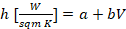, where a = 5.8 and b = 3.95.  I’ll give you wind speed data for Fort Collins, 2015, because the panel is roof mounted, allow convection only on the top of the panel.

·         The panel output power is a function of the solar input irradiance (I’ll give you irradiance data for Fort Collins, 2015), and the panel temperature.  The panel power is directly proportional to flat panel irradiance (Irradiancepanel), where 1000W/m2 solar irradiance input = 345W electrical output.  The spec sheet also describes includes a temperature coefficient (Pmpp) to derate the power production of the panel for each degree of temperature over 25C.

·         Given, solar elevation (same as solar altitude, a) and solar azimuth (A), you can calculate the incident radiation (Irradiancepanel) on a flat panel that has an orientation of azimuthal rotation (g) and tilt (b).  Our assumptions will be that the panel characteristics will be fixed in time (g,b), and that the solar coordinates (a,A) will change as a function of the time of day and of time of the year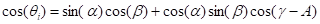Figure 3 is a diagram showing solar angles.  Note that panel azimuth (g) is defined in the same orientation as solar azimuth, and that panel tilt (b) is defined such that a horizontal panel on flat ground has b=0deg, and a vertical panel on a wall has b=90deg.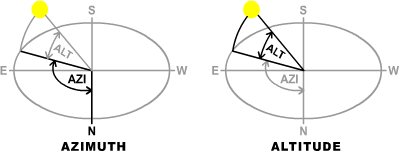Figure 3. Solar Angles

·         Type >>load solar_weather_data.mat , to load two arrays of data which are specific to Fort Collins, CO.

The first is “TimeDhiDniTempWindAzEl”, the columns of which are:

1.      Time [sec] of the year 2015

2.      Direct Horizontal Irradiation (not used)

3.      Direct Normal Irradiation [W/m2] – the solar power per unit area that strikes a plane pointed directly at the sun

4.      Temperature [C] – the ambient air temperature for 2015

5.      Wind Speed [m/s] – the wind speed for 2015

6.      Azimuth [degrees] – the solar azimuth (A) for 2015

7.      Elevation [degrees] – the solar elevation (a) for 2015

The second is “tou_rate_cents_per_kWh”, the columns of which are:

1.      Time [sec] of the year 2015

2.      Electricity Price [Cents/kWh electricity] – the time of use cost of electricity 

Problem 2) Compare the difference in value accrued over the entire year (365days/yr*24hrs/day*3600 sec/hr) between these two scenarios of PV system mounting.

1.      Mounting the panel directly to the roof, where only 1 side of the panel is cooled by the convection to the ambient air.

2.      Mounting the panel on a rack to the roof, where both sides of the panel is cooled by convection to the ambient air.  This can be modeled by considering the area of convection to be 2x the area considered above.

How much money could the racking system cost (per panel) to realize value for the PV system?

Problem 3) Assuming that I could mount the PV panel at any orientation (A, a) what is the optimal orientation that realizes maximum value from our PV system?  Getting the data in and out of Simulink can be a bit tricky, here is my augmented cost function:

function cost = augmented_cost_fxn(Az_Beta_inputs)

Az_input = Az_Beta_inputs(1);

Beta_input = Az_Beta_inputs(2);

simOut = sim('InsolationSimulation','SrcWorkspace','current');

cost = -simOut.get('dollars');  % make it negative for minimzation

end

And after some initializations, here is my function that calls fminsearch.

optim_options = optimset('Display','iter');

[x] = fminsearch('augmented_cost_fxn',[Az_input_init Beta_input_init], optim_options)

The rule of thumb is that facing direct south and at a tilt equal to the latitude of location is optimal.  Explain why the rule of thumb for PV orientation is wrong.  Explain why the rooftop PV market in Colorado will be approximately 50X smaller than the market in California.  Here is a clue.

Problem 4) You can see that the panel can reach over the 85C maximum temperature if it is not rack mounted.  Make the convection only happen on the top side of the panel, and add a constraint so that the panel never reaches over 85C.  What is the optimal orientation (A, a) that results in the temperature of the panel never going over 85C?  Plot temperature to verify that the constraint is met.

Writeup due in class before 11:59am Friday Dec 2.

Other stuff to do in preparation for the Lab of 11/28:

1)     Please break into groups of 4 students, exchange emails and cell numbers

2)     Designate one student with a well-functioning PC laptop

3)     On that computer, install a full version of MATLAB using the instructions below.  I have procured for you a full version of MATLAB with every possible toolset and option.  This is worth like \$25,000 per license and ensures that you have an ideal simulation environment, fully removed from ENS.  You are welcome to install this version of MATLAB on your personal computer.  Please do not take more than 1 license per group, do not sell or abuse the license; it is intended for coursework only.

To install the license, follow these steps using the computer you intend to use for your coursework.

User id: ..., pwd: H2e2going

4.      When prompted, choose Install using the Internet.  Select License 959549… Choose Typical Installation

4)     Download and install the MotoTune and MotoServer Software by searching for “mototune” at http://www.woodward.com/software.aspx.  You will have to register with Woodward’s website to get access.

5)     Download and install a GCC compiler by searching for “GCC PowerPC eabi SPE 4.6.0”.

http://www.engr.colostate.edu/~thb/Publications/MotoHawk-Release-2015a_sp0.1432.exe

 Applied Thermal Engineering Volume 28, Issues 8-9, June 2008, Pages 801-808

 http://www.powerfromthesun.net/Book/chapter04/chapter04.html

 http://www.pge.com/residentialtou/ , the E7 rate is the default residential time of use rate, comparable to the Xcel Energy rate referenced above

## Assignment DescriptionMECH 529 – Laboratory 11

“Optimization of Dynamic Systems”

(970) 491-3539, ...

Introduction: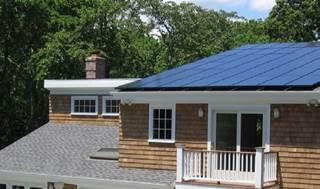All last week we have been talking about   methods         of         optimization of dynamic systems.  We now get the opportunity to apply that knowledge to our models.  Our application will be the power production of photovoltaic panels.

Learning Objectives:

1)     Test a number of the computer Figure 1. A sub-optimal PV installation modeling techniques that we have learned in the ME529 laboratory with the construction of an model of a nonlinear dynamic (thermal) system

2)     Introduce penalty functions as a means for imposing constraint on system optimization algorithms

3)     Use optimization to define ideal conditions of operation for a dynamic system

Many optimization routines (including Nelder Mead simplex) that are used for system design are unconstrained optimization routines.  To add constraints on the variables (that are changing during the optimization) requires an augmentation of the cost function.  One way to accomplish that is penalty functions.

As an example, let us assume that you would like to conduct an optimization for the problem:

### Minimize f(x)=-2x, subject to x<5

The problem is that Nelder-Mead has no way to incorporate your constraint on the admissible values of x.  You must augment the cost function f(x) with a penalty function that communicates violation of the constraint to the solver by increasing the cost function when the constraint is violated.  In this case, you can use a penalty function of the form:

### p(x) = rp*(max(0,x-5))2

The variable rp r is a variable that determines the size of the penalty that is applied when the constraint is violated.  As rp increases, violation of the constraints is more and more penalized.  Often you will change rp in subsequent optimization operations to assure that the constraints are not violated.  Addition of this penalty function to the cost function results in an augmented cost function of:

f(x)=2x + rp*(max(0,x-5))2

To construct a penalty function is easy for inequality constraints: put the constraint equation into the form of an equation that is less than zero (g(x)<0) and use rp*max(0,g(x))2 as the penalty function.  To construct a penalty function for equality constraints, put the constraint in the form of g(x)=0, and use the penalty function rp*g(x)2

Try to construct a penalty function with the following constraints:

### x=4

-x > 3 x1 <x2+5

One thing to note is that in order for the penalty functions to be effective, they must be of the same order of magnitude as each other and of the same order of magnitude (or greater) as the cost function.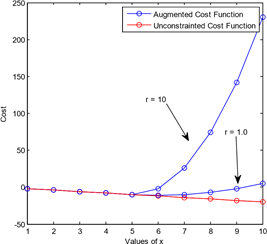Figure 2. Comparison of augmented and conventional cost function for the  external penalty method example problem, r=10

MATLAB™’s Nelder Mead n-dimensional simplex method is called fminsearch.  To do an example problem with fminsearch

Minimize        f = -x1x2

Subject to       g1 = x1+x22-1 < 0

g2 = -x1-x2 <0

To solve this problem you will have to make two m-files.  One will be a script file that you use to call fminsearch.  The other will be a function that evaluates f as a function of x1 and x2.  I pass the vector of x1 and x2 to the evaluation function along with the value of the penalty, r.  Here is the code for the script file.  I have varied r so that the constraints are:

clear close all

optim_options = optimset('Display','iter'); [x1,x2] = meshgrid(-1:.1:1, -1:.1:2); index = 0; for rp = [1:10:31] close all index=index+1;

f_const = -x1.*x2 + rp*max(0,x1+x2.^2-1).^2 + rp*max(0,-x1-x2).^2; f_unconst = -x1.*x2 ; subplot(1,2,1);

contourf(x1,x2, f_unconst,[-.5 -.4 [0:.5:5]]); title(' Unconstrained Problem'); colorbar subplot(1,2,2); contourf(x1,x2, f_const,[-.5 -.4 [0:.5:5]]); hold on ; colorbar plot([-1:.01:1],sqrt(-[-1:.01:1]+1),'k'); plot([-1 1], [1 -1],'k') title(['Find Optimum Between the 2 Black Lines, Rp = ' num2str(rp)]) [x] = fminsearch(@(x) example_fxn(x,rp),[-.5 -.5],optim_options)

Solution(index,:)=x;

end

Here is the code for the function.  You can see that I have put the penalty functions in the evaluation of the cost function f:

function f = example_fxn(x,rp) pause(.5)

f = -x(1)*x(2) + rp*max(0,x(1)+x(2)^2-1)^2 + rp*max(0,-x(1)-x(2))^2; plot(x(1), x(2),'ro-');

Run the example and watch the operation of the simplex algorithm.  You will see it accelerate towards the optimum when the slope is large.  The algorithm then refines estimations of the optimum as it gets closer and closer.  With rp = 1, the constraint is not very well enforced and the final value of x = (0.89, 0.67), outside the constraint.  With rp = 31, the constraint is well enforced and the final value of x = (0.67, 0.58).  Note that this is still just outside of the actual constraint (zoom in to see).  As rp gets bigger you see the constraints form a “bathtub” in which the optimizer can operate.

Task 3 – Thermal Photovoltaic Panel Model:

Dynamic Model

A photovoltaic (PV) panel generates electricity from the sun, but there are a number of consideration that determine how much value it will generate.  Its orientation, its temperature, and the time varying price of electricity; all determine what the value of the panel will be.  For this homework, we will compare a couple of options for configuring and orienting a PV system to maximize the economic value of the array.

Problem 1) Simulate the thermal behavior of this single solar photovoltaic panel over the first day (24*60*60 seconds) of the year.  Model the solar panel as a thermal capacitance (using the spec sheet value for mpanel and a referenced value for cp from glass) and a thermal resistance (due to convection to the ambient air).  With your system dynamics model you should be able to calculate the temperature of the panel as a function of time.

The global goal is to maximize the amount of money that your solar panel can earn.  You will have to construct a cost function to sum the amount of money that your solar panel will produce.

My house allows the panels to be mounted to the roof and oriented at A=180 degrees (pointing direct South) and β = 23deg (equal to my roof pitch).  Use those values for this initial simulation.

Please calculate the value that your panel can accrue over the 24 hours of Jan 1,

2015.  Plot a variety of dynamic inputs and states to cursorily validate your simulation.

Some important considerations are:

The ambient air temperature changes over the course of the day, I’ll give you the data for Fort Collins, 2015.

Wind tunnel tests show that the convection coefficient for a flat panel is a

𝑊𝑊 function of wind speed with a correlation relation : ℎ [  ] = 𝑎𝑎 + 𝑏𝑏𝑏𝑏,

𝑠𝑠𝑠𝑠𝑠𝑠 𝐾𝐾

where a = 5.8 and b = 3.95.  I’ll give you wind speed data for Fort Collins, 2015, because the panel is roof mounted, allow convection only on the top of the panel.

The panel output power is a function of the solar input irradiance (I’ll give you irradiance data for Fort Collins, 2015), and the panel temperature.  The panel power is directly proportional to flat panel irradiance (Irradiancepanel), where 1000W/m solar irradiance input = 345W electrical output.  The spec sheet also describes includes a temperature coefficient (Pmpp) to derate the power production of the panel for each degree of temperature over 25C.

Given, solar elevation (same as solar altitude, α) and solar azimuth (A), you can calculate the incident radiation (Irradiancepanel) on a flat panel that has an orientation of azimuthal rotation (γ) and tilt (β).  Our assumptions will be that the panel characteristics will be fixed in time (γ,β), and that the solar coordinates (α,A) will change as a function of the time of day and of time of the year2.

Irradiancepanel[W/m2]=DNI[W/m2] * cos(θi) cos(θi ) = sin(α)cos(β) + cos(α)sin(β)cos(γA)

Figure 3 is a diagram showing solar angles.  Note that panel azimuth (γ) is defined in the same orientation as solar azimuth, and that panel tilt (β) is defined such that a horizontal panel on flat ground has β=0deg, and a vertical panel on a wall has β=90deg.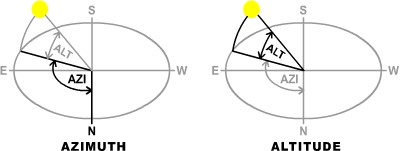Figure 3. Solar Angles

Type >>load solar_weather_data.mat , to load two arrays of data which are specific to Fort Collins, CO.

The first is “TimeDhiDniTempWindAzEl”, the columns of which are:

1.      Time [sec] of the year 2015

2.      Direct Horizontal Irradiation (not used)

3.      Direct Normal Irradiation [W/m2] – the solar power per unit area that strikes a plane pointed directly at the sun

4.      Temperature [C] – the ambient air temperature for 2015

5.      Wind Speed [m/s] – the wind speed for 2015

6.      Azimuth [degrees] – the solar azimuth (A) for 2015

7.      Elevation [degrees] – the solar elevation (α) for 2015 The second is “tou_rate_cents_per_kWh”, the columns of which are:

1.      Time [sec] of the year 2015

2.      Electricity Price [Cents/kWh electricity] – the time of use cost of electricity 

Problem 2) Compare the difference in value accrued over the entire year (365days/yr*24hrs/day*3600 sec/hr) between these two scenarios of PV system mounting.

1.      Mounting the panel directly to the roof, where only 1 side of the panel is cooled by the convection to the ambient air.

2.      Mounting the panel on a rack to the roof, where both sides of the panel is cooled by convection to the ambient air.  This can be modeled by considering the area of convection to be 2x the area considered above.

How much money could the racking system cost (per panel) to realize value for the PV system?

Problem 3) Assuming that I could mount the PV panel at any orientation (A, α) what is the optimal orientation that realizes maximum value from our PV system?6  Getting the data in and out of Simulink can be a bit tricky, here is my augmented cost function:

function cost = augmented_cost_fxn(Az_Beta_inputs)

load solar_weather_data      Az_input = Az_Beta_inputs(1);     Beta_input = Az_Beta_inputs(2);

cost = -simOut.get('dollars');  % make it negative for minimzation end

And after some initializations, here is my function that calls fminsearch.

optim_options = optimset('Display','iter');

[x] = fminsearch('augmented_cost_fxn',[Az_input_init Beta_input_init], optim_options)

The rule of thumb is that facing direct south and at a tilt equal to the latitude of location is optimal.  Explain why the rule of thumb for PV orientation is wrong.  Explain why the rooftop PV market in Colorado will be approximately 50X smaller than the market in California.  Here is a clue.

Problem 4) You can see that the panel can reach over the 85C maximum temperature if it is not rack mounted.  Make the convection only happen on the top side of the panel, and add a constraint so that the panel never reaches over 85C.  What is the optimal orientation (A, α) that results in the temperature of the panel never going over 85C?  Plot temperature to verify that the constraint is met.

Writeup due in class before 11:59am Friday Dec 2.

Other stuff to do in preparation for the Lab of 11/28:

1)     Please break into groups of 4 students, exchange emails and cell numbers

2)     Designate one student with a well-functioning PC laptop

3)     On that computer, install a full version of MATLAB using the instructions below.  I have procured for you a full version of MATLAB with every possible toolset and option.  This is worth like \$25,000 per license and ensures that you have an ideal simulation environment, fully removed from ENS.  You are welcome to install this version of MATLAB on your personal computer.  Please do not take more than 1 license per group, do not sell or abuse the license; it is intended for coursework only.

To install the license, follow these steps using the computer you intend to use for your coursework.

User id: ..., pwd: H2e2going

4.      When prompted, choose Install using the Internet.  Select License 959549… Choose Typical Installation

4)     Download and install the MotoTune and MotoServer Software by searching for “mototune” at http://www.woodward.com/software.aspx.  You will have to register with Woodward’s website to get access.

5)     Download and install a GCC compiler by searching for “GCC PowerPC eabi SPE

4.6.0”.

http://www.engr.colostate.edu/~thb/Publications/MotoHawk-Release-

 Applied Thermal Engineering Volume 28, Issues 8-9, June 2008, Pages 801-808

 http://www.powerfromthesun.net/Book/chapter04/chapter04.html

 http://www.pge.com/residentialtou/ , the E7 rate is the default residential time of use rate, comparable to the Xcel Energy rate referenced above

Is it free to get my assignment evaluated?

Yes. No hidden fees. You pay for the solution only, and all the explanations about how to run it are included in the price. It takes up to 24 hours to get a quote from an expert. In some cases, we can help you faster if an expert is available, but you should always order in advance to avoid the risks. You can place a new order here.

How much does it cost?

The cost depends on many factors: how far away the deadline is, how hard/big the task is, if it is code only or a report, etc. We try to give rough estimates here, but it is just for orientation (in USD):

 Regular homework \$20 - \$150 Advanced homework \$100 - \$300 Group project or a report \$200 - \$500 Mid-term or final project \$200 - \$800 Live exam help \$100 - \$300 Full thesis \$1000 - \$3000

How do I pay?

Credit card or PayPal. You don't need to create/have a Payal account in order to pay by a credit card. Paypal offers you "buyer's protection" in case of any issues.

Why do I need to pay in advance?

We have no way to request money after we send you the solution. PayPal works as a middleman, which protects you in case of any disputes, so you should feel safe paying using PayPal.

Do you do essays?

No, unless it is a data analysis essay or report. This is because essays are very personal and it is easy to see when they are written by another person. This is not the case with math and programming.

Why there are no discounts?

It is because we don't want to lie - in such services no discount can be set in advance because we set the price knowing that there is a discount. For example, if we wanted to ask for \$100, we could tell that the price is \$200 and because you are special, we can do a 50% discount. It is the way all scam websites operate. We set honest prices instead, so there is no need for fake discounts.

Do you do live tutoring?

No, it is simply not how we operate. How often do you meet a great programmer who is also a great speaker? Rarely. It is why we encourage our experts to write down explanations instead of having a live call. It is often enough to get you started - analyzing and running the solutions is a big part of learning.

What happens if I am not satisfied with the solution?

Another expert will review the task, and if your claim is reasonable - we refund the payment and often block the freelancer from our platform. Because we are so harsh with our experts - the ones working with us are very trustworthy to deliver high-quality assignment solutions on time.

Customer Feedback

"Thanks for explanations after the assignment was already completed... Emily is such a nice tutor! "

Order #13073